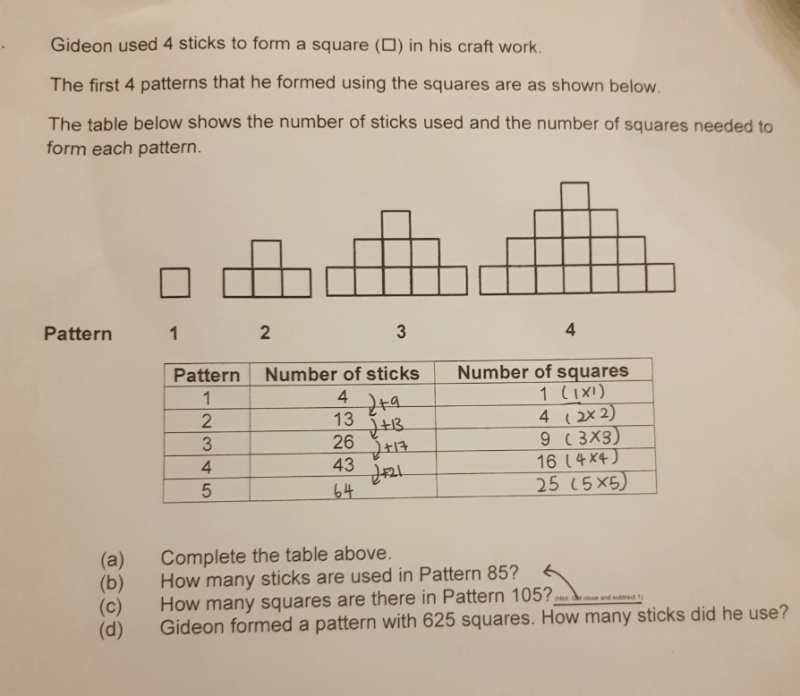# QuestionPlease explain how to find the pattern. Thanks.

1 Answer

# Answer

 Pattern No. of Sticks 1 4 4 × (1) + 0 2 13 4 × (1 + 2) + 1 3 26 4 × (1 + 2 + 3) + 2 4 43 4 × (1 + 2 + 3  + 4) + 3 5 64 4 × (1 + 2 + 3  + 4 + 5) + 4 n 4 × (1 + 2 + 3  +…..+ n) + (n – 1)

Formula for (1 + 2 + 3  +…..+ n) is n(n + 1) /2

So formula for number of sticks is 4n(n + 1)/2 + (n – 1) or 2n(n + 1) + (n – 1)

0 Replies 0 Likes